# Transport Phenomena

What exactly are "transport phenomena"?

Transport phenomena are really just a fancy way that Chemical Engineers group together three areas of study that have certain ideas in common. These three areas of study are:

• Fluid Mechanics
• Heat Transfer
• Mass Transfer

Why do people group these together?

Well, they are all the study of the transfer of something. Fluid Mechanics deals with the transfer of momentum in a fluid. Heat Transfer deals with the transfer of heat, and Mass Transfer deals with the transfer of mass.

What does that mean, though?

Fluid Mechanics deals with the transfer of momentum in a fluid. On a molecular scale that means that the molecules banging into each other transfer their momentum to other molecules. On a larger scale, these molecules banging into each other determine how the fluid is going to flow-that is, if it will just flow smoothly (or 'laminar'), or if it will be rough (or 'turbulent').

Heat Transfer deals with the transfer of heat. Heat is just another way to say energy. So, really we are studying how energy moves around. Typical methods of Heat Transfer are:

• Conduction. If you touch something hot, you get heat transfer via conduction.
• Convection. If you hold your hand above a burner on a stove, the hot air rising from the burner is moving heat via convection.
• Radiation. Stand in the sun. Now, move to the shade. The warmth that you feel from the sun is heat transferred via radiation.

Mass Transfer deals with the transfer of mass. One example of mass transfer is if you take a glass of water and put one drop of red dye in it. Even if you don't stir the water, eventually the water is all the same pinkish color. The study of how the dye spreads out is Mass Transfer.

OK, then how are they all related?

They all are similar in their behavior. They all move stuff (Momentum, Heat, or Mass) from a place where there is a lot of the stuff to a place where there is less stuff. Here are some examples:

In Fluid Mechanics momentum is transferred from a place where we have a lot of momentum to a place where we have less. A good analogy is the flow of traffic on a busy freeway. The far right lane on the freeway typically move slower than the far left lane, with the lanes in the middle going faster the further left you move. This can be compared to flow over a flat plate, where the slower flow (the right lane on the freeway) is right next to the plate, and the faster flow (the left lane on the freeway) on the surface of the fluid. The transfer of momentum is like the cars changing lanes, as slower cars pull into faster lanes the lanes slow down to allow the car to accelerate (and not cause a pile-up on the freeway), and the faster cars pulling into slower lanes and speeding up the lane a little bit.

In Heat Transfer, energy moves from a place where there is a lot to a place where there is less. For example, if you heat up a brick, then drop it into cold water, the brick gets colder and the water gets warmer. Once the brick and the water are at the same temperature, no more energy can be transferred.

Mass Transfer. Back to the red dye example: the red dye when it is first dropped into the water is at a high concentration and the water is at zero concentration. But, as the dye spreads out, the concentration of the dye slowly increases, until, it is all at the same low concentration everywhere. Once the concentration of the dye in the water is the same everywhere, no more mass transfer can take place.

The one of the most important similarities between all of these examples is: There is a driving force (momentum, temperature, or concentration difference or 'gradient'), which becomes smaller as time progresses in each of the examples, and eventually becomes zero when no more transfer of stuff takes place.

Give me the math!

The math for all of these "transport phenomena" all are based on 2 ideas:

• The rate of change of stuff is proportional to some driving force, as in the examples above.
• We can't destroy mass or energy (or, mass and energy must be conserved).

Driving Force Equations

The first idea is summed up by three similar laws for each of the three "transport phenomena" (here in one dimension and rectangular coordinates):

• Newton's Law, fluid mechanics:
•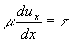where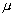= viscosity of fluid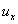= velocity of fluid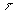= sheer stress on the fluid

• Fourier's Law, heat transfer:
•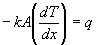where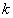= thermal conductivity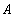= area for heat transfer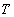= temperature= heat flow per unit time

• Fick's Law, mass transfer: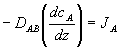where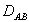= diffusivity of A in B= concentration of A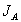= molar flux with respect to molar average velocity

Conservation of Mass and Energy

The idea behind the conservation of mass and energy can be thought of a little like a shoe box: if you put stuff into it, you can either take it out or leave it in the box, you can't make the stuff disappear. Or, in the form of an equation:

stuff in - stuff out = stuff still in the box (or accumulation)

This idea can be summed for Fluid Mechanics by the use of the Continuity Equation (which uses the idea of the conservation of mass) and the Navier-Stokes Equation (which uses the idea of the conservation of momentum).

It should be noted that these equations are typically simplified before they are solved.

• Continuity Equationwhere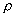= density of the fluid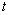= time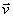= velocity vector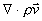=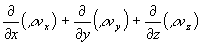in rectangular coordinates

• Navier-Stokes Equation in rectangular coordinates

X component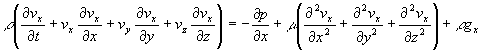Y component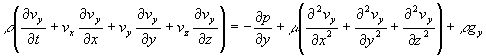Z component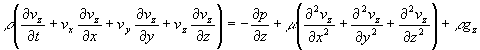For Heat Transfer and Mass Transfer there are similar equations that are based on the conservation of mass and energy.

 Home I<= Back <= Next =>